Search by Topic

Resources tagged with Investigations similar to Ideal Gases:

Filter by: Content type:
Age range:
Challenge level:

There are 57 results

Broad Topics > Using, Applying and Reasoning about Mathematics > Investigations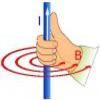Electromagnetism

Age 16 to 18

Read all about electromagnetism in our interactive article.Escape from Planet Earth

Age 16 to 18 Challenge Level:

How fast would you have to throw a ball upwards so that it would never land?Modelling Assumptions in Mechanics

Age 16 to 18

An article demonstrating mathematically how various physical modelling assumptions affect the solution to the seemingly simple problem of the projectile.Powerfully Fast

Age 16 to 18 Challenge Level:

Explore the power of aeroplanes, spaceships and horses.Bessel's Equation

Age 16 to 18 Challenge Level:

Get further into power series using the fascinating Bessel's equation.The Power of Dimensional Analysis

Age 14 to 18

An introduction to a useful tool to check the validity of an equation.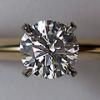Diamonds Aren't Forever

Age 16 to 18 Challenge Level:

Ever wondered what it would be like to vaporise a diamond? Find out inside...Mach Attack

Age 16 to 18 Challenge Level:

Have you got the Mach knack? Discover the mathematics behind exceeding the sound barrier.Big and Small Numbers in Physics

Age 14 to 16 Challenge Level:

Work out the numerical values for these physical quantities.Global Warming

Age 14 to 16 Challenge Level:

How much energy has gone into warming the planet?Big and Small Numbers in Chemistry

Age 14 to 16 Challenge Level:

Get some practice using big and small numbers in chemistry.Big and Small Numbers in the Physical World

Age 14 to 16 Challenge Level:

Work with numbers big and small to estimate and calculate various quantities in physical contexts.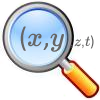A Different Differential Equation

Age 16 to 18 Challenge Level:

Explore the properties of this different sort of differential equation.Taking Trigonometry Series-ly

Age 16 to 18 Challenge Level:

Look at the advanced way of viewing sin and cos through their power series.Big and Small Numbers in the Living World

Age 11 to 16 Challenge Level:

Work with numbers big and small to estimate and calculate various quantities in biological contexts.Age 16 to 18

Read about the mathematics behind the measuring devices used in quantitative chemistryGenetic Intrigue

Age 16 to 18

Dip your toe into the fascinating topic of genetics. From Mendel's theories to some cutting edge experimental techniques, this article gives an insight into some of the processes underlying. . . .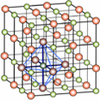Clear as Crystal

Age 16 to 18 Challenge Level:

Unearth the beautiful mathematics of symmetry whilst investigating the properties of crystal lattices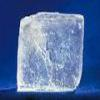What Salt?

Age 16 to 18 Challenge Level:

Can you deduce why common salt isn't NaCl_2?Smoke and Daggers

Age 16 to 18 Challenge Level:

We all know that smoking poses a long term health risk and has the potential to cause cancer. But what actually happens when you light up a cigarette, place it to your mouth, take a tidal breath. . . .Stirling Work

Age 16 to 18 Challenge Level:

See how enormously large quantities can cancel out to give a good approximation to the factorial function.Problem Solving: Opening up Problems

Age 5 to 16

All types of mathematical problems serve a useful purpose in mathematics teaching, but different types of problem will achieve different learning objectives. In generalmore open-ended problems have. . . .Scale Invariance

Age 16 to 18 Challenge Level:

By exploring the concept of scale invariance, find the probability that a random piece of real data begins with a 1.Making More Tracks

Age 16 to 18 Challenge Level:

Given the equation for the path followed by the back wheel of a bike, can you solve to find the equation followed by the front wheel?Building Approximations for Sin(x)

Age 16 to 18 Challenge Level:

Build up the concept of the Taylor series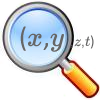Trig-trig

Age 14 to 18 Challenge Level:

Explore the properties of combinations of trig functions in this open investigation.Age 14 to 18 Challenge Level:

Some of our more advanced investigationsWhich Twin Is Older?

Age 16 to 18

A simplified account of special relativity and the twins paradox.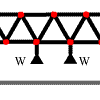More Bridge Building

Age 16 to 18 Challenge Level:

Which parts of these framework bridges are in tension and which parts are in compression?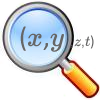Chance of That

Age 14 to 18 Challenge Level:

What's the chance of a pair of lists of numbers having sample correlation exactly equal to zero?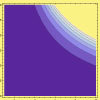Sextet

Age 16 to 18 Challenge Level:

Investigate x to the power n plus 1 over x to the power n when x plus 1 over x equals 1.Few and Far Between?

Age 14 to 18 Challenge Level:

Can you find some Pythagorean Triples where the two smaller numbers differ by 1?Perfect Eclipse

Age 14 to 16 Challenge Level:

Use trigonometry to determine whether solar eclipses on earth can be perfect.Very Old Man

Age 16 to 18 Challenge Level:

Is the age of this very old man statistically believable?Eight Ratios

Age 14 to 16 Challenge Level:

Two perpendicular lines lie across each other and the end points are joined to form a quadrilateral. Eight ratios are defined, three are given but five need to be found.The Invertible Trefoil

Age 14 to 16 Challenge Level:

When is a knot invertible ?9 Hole Light Golf

Age 5 to 18 Challenge Level:

We think this 3x3 version of the game is often harder than the 5x5 version. Do you agree? If so, why do you think that might be?Designing Table Mats

Age 11 to 16 Challenge Level:

Formulate and investigate a simple mathematical model for the design of a table mat.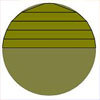Peeling the Apple or the Cone That Lost Its Head

Age 14 to 16 Challenge Level:

How much peel does an apple have?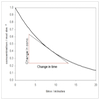Reaction Rates!

Age 16 to 18

Fancy learning a bit more about rates of reaction, but don't know where to look? Come inside and find out more...A Rational Search

Age 14 to 18 Challenge Level:

Investigate constructible images which contain rational areas.Two Regular Polygons

Age 14 to 16 Challenge Level:

Two polygons fit together so that the exterior angle at each end of their shared side is 81 degrees. If both shapes now have to be regular could the angle still be 81 degrees?What Do Functions Do for Tiny X?

Age 16 to 18 Challenge Level:

Looking at small values of functions. Motivating the existence of the Taylor expansion.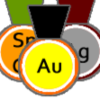Alternative Record Book

Age 14 to 18 Challenge Level:

In which Olympic event does a human travel fastest? Decide which events to include in your Alternative Record Book.Geometry and Gravity 1

Age 11 to 18

This article (the first of two) contains ideas for investigations. Space-time, the curvature of space and topology are introduced with some fascinating problems to explore.CSI: Chemical Scene Investigation

Age 16 to 18 Challenge Level:

There has been a murder on the Stevenson estate. Use your analytical chemistry skills to assess the crime scene and identify the cause of death...Carbon Footprints

Age 14 to 16 Challenge Level:

Is it really greener to go on the bus, or to buy local?Robot Camera

Age 14 to 16 Challenge Level:

Could nanotechnology be used to see if an artery is blocked? Or is this just science fiction?Bent Out of Shape

Age 14 to 18 Challenge Level:

An introduction to bond angle geometry.Spirostars

Age 16 to 18 Challenge Level:

A spiropath is a sequence of connected line segments end to end taking different directions. The same spiropath is iterated. When does it cycle and when does it go on indefinitely?